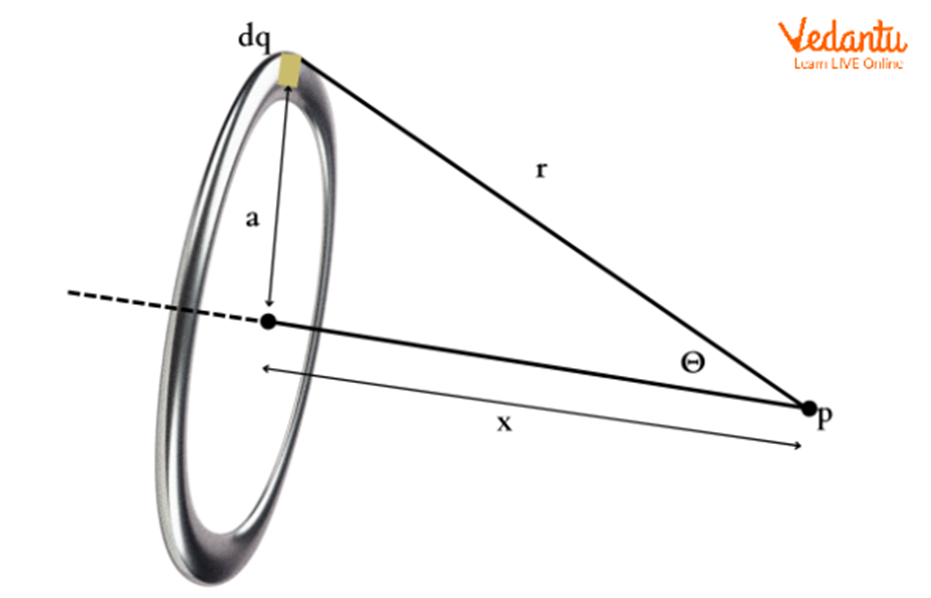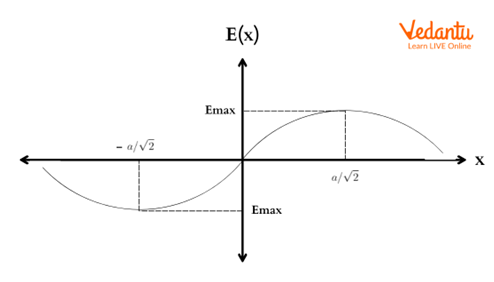Courses
Courses for Kids
Free study material
Free LIVE classes
More

# Electric Field Due To a Uniformly Charged Ring - JEELIVE
Join Vedantu’s FREE Mastercalss

## What is the Significance of the Axis of a Charged Ring?

An electric field gets associated with any charged point in space. Such an electric field is often quantified using terms such as the electric field strength and intensity. The electric field can be present in any object irrespective of how the charge is distributed.

For instance, an electric field gets created along with the spherical, linear, and planar distribution of charges. In all the above cases, the electric field has a magnitude and a direction. Likewise, when a ring is charged either uniformly or non-uniformly, an electric field gets created along the axis of the ring, the magnitude, and direction of which gets determined by the charge on the ring itself.

In this article, you will learn about the axis of a uniformly charged ring and the electric field due to the ring. It is significant to understand the nature and magnitude of the electric field at various points along the axis to understand the force it would exert on any unit positive charge kept nearby.

## Electric Field due to Arc of Ring

An electric field around any charge distribution can be found by creating an element out of infinitesimal point charges. In the case of a uniformly charged ring, the electric field on the axis of a ring, which is uniformly charged, can be found by superimposing the electric fields of an infinitesimal number of charged points. The ring is then treated as an element to derive the electric field of a uniformly charged disc.

### Deriving the Circular Ring Formula:

Consider the following figure as a charged ring whose axis is subjected to an electric field of varying intensity from the centre of the charged ring.Electric Field at P due to an Infinitesimal Charge dq on a Charged Ring

Let’s now derive the equation to find the electric field along the axis at a distance of x from the centre of the charged ring. Here, $r = \sqrt{x^{2} + a^{2}}$ is the distance of point p from the arc element dq.

According to the principle of superposition, the total electric field at point p (along the axis of the charged ring) is the vector sum of individual electric fields due to all the point charges.

According to Gauss Law, the electric field caused by a single point charge is as follows:

$\overrightarrow{E} = \dfrac {1}{4~\pi ~ \epsilon_0} \times {\dfrac {q}{r^2}}~\hat {r}$

The electric field at point p due to the small point charge dq which is at a radius of a from the centre of the charged ring can be written as:

$\overrightarrow{dE} = \dfrac {1}{4~\pi ~ \epsilon_0} \times {\dfrac {dq}{r^2}}~\hat {r}$

It is important to note that since there is a corresponding piece of point charge on the opposite side of the ring, the y components of the electric field will get nulled throughout.

Hence, only the x component of the electric field will be significant in deriving the total electric field at point p due to a charged ring.

However, we can see that the tiny x component forms an angle Θ at point p along the axis of the charged ring. Hence the electric field equation will be adjusted while considering this angle and hence becomes:

$dE_{x} = \dfrac {1}{4~\pi ~ \epsilon_0} \times {\dfrac {q}{r^2}}~\cos \Theta$

Since the axis forms a right angle with the distance from dq to point p and Θ is unknown, we replace r and $\cos \Theta$ with the known distances x and a. The above equation thus becomes:

$dE_{x} = \dfrac {1}{4~\pi ~ \epsilon_0} \times {\dfrac {dq}{\left({x^2}+{a^2}\right)}}~{\dfrac {x}{\sqrt {\left({x^2}+{a^2}\right)}}}$

Upon simplification,

$dE_{x} = \dfrac {1}{4~\pi ~ \epsilon_0} \times {\dfrac {x~dq}{\left({x^2}+{a^2}\right)^{\dfrac {3}{2}}}}$

Now, by integrating the above equation with respect to dq, the total electric field at point p on the axis of a charged ring is given by the following equation:

$E_{x}=\dfrac {1}{4~\pi ~ \epsilon_0} \times {\dfrac {x~Q}{\left({x^2}+{a^2}\right)^{\dfrac {3}{2}}}}$

Note: The above equation holds good only for finding the electric field on any point on the x-axis.

Let us now draw a graph that closely represents the relationship between the electric field along the axis of a charged ring and the distance from the centre of the charged ring.

The electric field is zero at the centre and increases to a maximum on either side of the ring, and then gradually falls back to zero as x approaches infinity. The magnitude of the electric field will be the same due to symmetry and uniformity in the distribution of charge, and the closest graphical representation or the graph of a uniformly charged ring is drawn below:Electric Field Intensity along the Axis of a Charged Ring

## Key Points for JEE Exams

• When the point p is very far from the centre of the charged ring i.e., x >> a, then the electric field equation is the same as that due to a point charge, as a is very insignificant such that it becomes zero. Hence, the electric field equation when x >> a is

$E_{x} = (Q/4\pi \epsilon _{o}x^{2} )$

• When the point p is at the centre of the ring, x = 0. Hence the electric field at the centre of a charged ring is zero which is in conformance with symmetry and uniformity.

• The maximum possible electric field intensity can be derived using dE/dx = 0. In this case, it is observed that the maximum electric field strength occurs when  $\pm a\sqrt{2}$ and is given by the following equation:

$E_{max} = Q/6\sqrt{3}\pi \epsilon a^{2}$

• The magnetic field due to the ring is $B=2\left( R+x \right)\mu lx$.

## Conclusion

The charge distribution along the axis of an electrically charged ring will be symmetric on either side of the ring, and, hence, the electric field will be in a direction that is along the axis of the charged ring. The resultant electric field at the centre of the ring will be zero, and it will increase to a maximum at a distance of $a/\sqrt{2}$ on either side of the charged ring. Then, at an infinite distance from the centre, the electric field becomes zero again.

Last updated date: 19th Sep 2023
Total views: 117.3k
Views today: 2.17k

## FAQs on Electric Field Due To a Uniformly Charged Ring - JEE

1. Does a ring charge behave like a point charge? If so, when does this happen?

Yes. A charged ring will behave like a point charge when the distance from the point p to the centre of the charged ring exceeds the radius of the charge distribution to a greater extent. In that case, the radius is negligible and the charge distribution starts to act as a single point charge i.e., at such a far away distance, a ring charge is perceived as a point charge.

The electric field intensity in such a case will be $E_{x} = \dfrac {Q}{4~\pi ~ \epsilon _{o}~{x^2}}$.

2. What is a uniformly charged ring?

A uniformly charged ring is the one in which the charge is distributed uniformly along the circumference of the ring. The uniformly charged ring plays an important role in understanding electric field generation.